Nivethitha

updated on 07-03-2023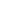# CGPA to percentage## CGPA Calculator: Convert CGPA to percentage

Percentage

Nowadays the academic performance of graduate students is calculated using grade points. The universities have started using the CGPA and SGPA methods to calculate their student's results. This result can be converted from CGPA to percentage using the basic formula. The students can also quickly determine their final percentage using the CGPA to percentage calculator that is available online.

In this article, let us see how to convert the CGPA to percentage using the formula, convert percentage to CGPA, etc. Here we have also discussed the CGPA to Percentage calculation in different Indian universities. This article will guide you in gaining a clear understanding of the latest grading system used in our education institutions.

## What is CGPA?

Cumulative Grade Point Average (CGPA) is an educational grading system used in schools and colleges to measure students' overall academic performance. The CGPA is calculated by taking the average of all the grade points obtained in each semester or SGPA in their entire study tenure.

The CGPA is calculated for all the academic subjects omitting the supplementary course work completed during the education period. This can be easily converted into a percentage using the simple CGPA to percentage conversion formula. The CGPA is used by universities across the globe to offer admission to their institutions.

## Benefits of the CGPA systemThe advantages of using the CGPA by education institutions are given below.

• Learn without any pressure: This scoring pattern has relieved the exam stress of the students as there is no particular emphasis on the marks obtained.
• using the grades and calculating the overall performance based on the grades obtained indicates the advancement in the marking system.
• Clear insight into the student's performance: The individual subjects’ performance of the students can be easily interpreted based on the grades obtained. This will guide the teachers to aid them in their exam preparation.
• Identify the student's strengths and weaknesses: The student's performance can be easily identified and the necessary actions can be taken to improvise their scores.
• The education system is simplified: With the new method of scoring, the education system has become easier and simpler for students in many ways.

• The level of competition among the students is reduced as they are only awarded a cumulative grade. Some students may be demotivated as there is no need to score a higher mark.
• This marking system can be considered unfair, as the students securing 90 and 98 are given the same grade.
• The grades are considered to reward more percentage than the actually secured marks.
• The results may be inaccurate as the students scoring 89 and 90 are falling into two different grades and the difference between the two is immense. This may affect the overall students' performance.

## Difference between CGPA and GPA

The main difference between the CGPA and GPA is that GPA is the performance measure of the student for the individual semester and the CGPA is the overall performance measure of the students in their entire course duration.

The other differences between the CGPA and GPA are mentioned below for your reference:

• The GPA is calculated for a shorter duration i.e one semester. The CGPA is calculated for the entire course duration. (i.e for the Engineering course, CGPA is calculated for 4 years - 8 semesters)
• The GPA of the individual semesters affects the overall CGPA as the latter is calculated based on the GPA. Thus a good GPA is necessary.
• International universities usually consider GPA for their admission eligibility as it will showcase the students’ performance throughout the course.
• The 10-point CGPA grading system is used by Indian Universities, whereas the 4-point GPA is used by UK institutions.
• CBSE board has a curriculum for CGPA calculation but not for GPA calculation.

## How to calculate CGPA?

The CGPA is calculated by dividing the total grade points obtained in all major subjects by the total credit points. The CGPA ranges from 0.00 to 10.00 and will be expressed using two decimal points.

 CGPA = ∑ (Ci*GPi)/ ∑ Ci WhereCi- Credit Points GPi - Grade Points

## How to convert CGPA to percentage?

To convert CGPA to percentage, you can use the following steps.

• Step 1: Add the grade points of all the subjects in the course.
• Step 2: Add the credit points of all the subjects.
Step 3: Calculate the CGPA by dividing the summation of grade points by the summation of credit points.
• Step 4: Multiply the CGPA with 9.5 to obtain your overall percentage from CGPA.

The CGPA To percentage calculator can also be used. For your easy reference, we have given the CGPA to percentage calculation.

1. For instance, you have appeared for a 5 subject semester and the calculation is as follows, the scores obtained in the 5 subjects are:

Subject 1- 10
Subject 2- 8
Subject 3 - 9
Subject 4- 7
Subject 5- 8

CGPA = (10+8+9+8+7)/ 5
= 44/5
= 8.80

8.8 CGPA to percentage = CGPA*9.5
= 8.80 * 9.5
= 83.6%

2.For example, if you have scored the following grade points in your semester,
Chemistry - 6
Physics - 8
Mathematics - 7
Psychology - 7

Then your CGPA calculation will be as follows,

CGPA= (summation of the scores obtained in each subject)/ total number of subjects
= (6+8+7+7)/4
= 28/4
= 7.00

CGPA to percentage= 7.00*9.5
= 66.5%

## CGPA to Percentage Conversion Chart (9.5 Grading Scale)

Take a look at the CGPA to Percentage Conversion Chart, given below. We have converted ‘CGPA in percentage’ using the ‘CGPA*9.5’ formula. It is a calculation method of CBSE. You can check the equivalent percentage of your CGPA.

 CGPA Percentage (%) CGPA Percentage (%) 10 95% 6.9 65.55% 9.9 94.05% 6.8 64.6% 9.8 93.1% 6.7 63.65% 9.7 92.15% 6.6 62.7% 9.6 91.2% 6.5 61.75% 9.5 90.25% 6.4 60.8% 9.4 89.3% 6.3 59.85% 9.3 88.36% 6.2 58.9% 9.2 87.4% 6.1 57.95% 9.1 86.45% 6 57% 9 85.5% 5.9 56.05% 8.9 84.55% 5.8 55.1% 8.8 83.6% 5.7 54.15% 8.7 82.65% 5.6 53.2% 8.6 81.7% 5.5 52.25% 8.5 80.75% 5.4 51.3% 8.4 79.8% 5.3 50.35% 8.3 78.85% 5.2 49.4% 8.2 77.9% 5.1 48.45% 8.1 76.95% 5 47.5% 8 76% 4.9 46.56% 7.9 75.05% 4.8 45.6% 7.8 74.1% 4.7 44.65% 7.7 73.15% 4.6 43.7% 7.6 72.2% 4.5 42.75% 7.5 71.25% 4.4 41.8% 7.4 70.3% 4.3 40.85% 7.3 69.35% 4.2 39.9% 7.2 68.4% 4.1 38.95% 7.1 67.45% 4 38% 7 66.5%

The Grade table as per the CBSE is shared for your reference

 Marks CGPA Grades 91-100 10 A1 81-90 9 A2 71-80 8 B1 61-70 7 B2 51-60 6 C1 41-50 5 C2 33-40 4 D 21-32 0 E1 00-20 0 E2

## CGPA to percentage calculator for different Indian universities

CGPA grading system is widely used by universities in India to calculate the student's performance in each semester and in the entire course duration. Here we have given the CGPA to percentage calculation followed by a few of the top universities in the country.

### CBSE - CGPA to percentage calculation

To convert CGPA to percentage, the CBSE board is following the basic formula of multiplying the CGPA value by 9.5. This percentage calculation is used to identify the performance of the students in classes IX and X.

For instance, for 8 CGPA in percentage is 8*9.5 = 76%

The CBSE has revised the CGPA to percentage calculation for the convenience of the students.

Subject-wise percentage calculation = 9.5*GPA of the individual subject
Overall exam percentage calculation = 9.5* CGPA

### Anna University CGPA to percentage calculation

The Anna University CGPA to percentage calculation is applicable for Anna University and its affiliated colleges only. Here the percentage from CGPA will be obtained by multiplying the CGPA by 10.

Anna University CGPA to percentage conversion = CGPA *10

For example, if your CGPA is 7.6, then the percentage will be 76%.

Check out the Anna university grades, and credit points for the semester exams.

 Marks Grade Credit points >91 S 10 81-90 A 9 71-80 B 8 61-70 C 7 57-60 D 6 51-56 E 5 <50 U 0

### CGPA to percentage VTU calculation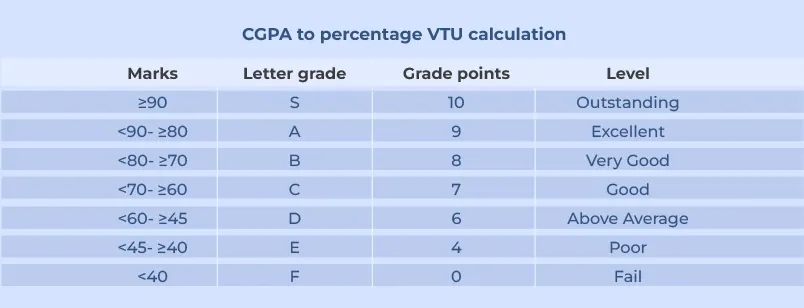In the Visvesvaraya Technological University there are two types of the scoring system. They are the Choice Based Credit System (CBCS) and Credit Based Semester System (CBSS).
The CGPA to Percentage VTU grading system is as follows.

The VTU grading system uses the SGPA and CGPA to measure the student's performance. The SGPA is the Semester Grade Point Average, indicating the performance of the students per semester. The SGPA can be converted to CGPA, using the formula

CGPA = ∑ (Ci*Si) / ∑ Ci

Where Ci is the Credit Points
Si is the SGPA

The CGPA to percentage VTU conversion = (CGPA-0.75) * 10

The CGPA from percentage can be calculated using the formula - (Aggregate percentage / 10) + 0.75

### KTU CGPA to percentage conversion

APJ Abdul Kalam Technological University or Kerala Technological University follows the 10-point Grade system. The results are provided on the basis of SGPA and CGPA. However, the KTU board has given a CGPA to the percentage conversion formula that is applicable only to KTU students. You can find the formula below for KTU CGPA to Percentage Conversion.

 KTU CGPA to Percentage = (10*Gi)- 3.75  Where G stands for CGPA or SGPA

### CGPA to percentage GTU conversion

The Gujarat Technological University has 2 years and 4 years of course. They follow the CGPA and CPT system of scoring. The obtained grades can be converted into percentages using the formula prescribed by the Board to be followed only by their students.

CGPA to percentage GTU formula = ((SPI \ CPI \ CGPA) – 0.5) * 10
Where
SPI- Semester Percentage Index
CPI- Cumulative Percentage Index

### CGPA to percentage SPPU Conversion

The SPPU follows the 10-point grade system. The performance of the students is measured using the CGPA and SGPA. The CGPA to percentage SPPU conversion formula is different for each grade point. As the marks obtained in the semesters play a vital role in further education and in job opportunities, the college wants to have a perfect scoring system and thus multiple formulas are used for the conversion.

The CGPA to percentage SPPU formulas is,

The CGPA to percentage conversion for Grade D (4- 4.75 CGPA)

Percentage = (CGPA*6.6)+13.5

CGPA to percentage calculation for Grade C (4.75 - 5.25 CGPA)

Percentage = (CGPA*10)-2.5

CGPA to percentage conversion for Grade B (5.25 - 5.75 CGPA)

Percentage = (CGPA*10)-2.5

CGPA to percentage calculation for Grade B= (5.75 - 6.75 CGPA)

Percentage = (CGPA*5)+26.25

Percentage from CGPA for Grade A (6.75 - 8.25 CGPA)

Percentage = (CGPA*10)-7.5

Percentage from CGPA for Grade A+ (8.25 - 9.50 CGPA)

Percentage = (CGPA*12)-25

Percentage from CGPA conversion for Grade O (9.50 - 10.00 CGPA)

Percentage = (CGPA*20)-100

### BPUT CGPA to percentage Conversion

The Biju Patnaik University of Technology has given its own CGPA to percentage calculation. This BPUT CGPA to Percentage conversion method is applicable only for their college students.

 BPUT CGPA to percentage = (CGPA-0.50)*10

### CGPA to percentage RGPV

RGPV used the credit-based scoring system. The conversion formula applicable for this university is multiplying the CGPA with 10.

RGPV CGPA to percentage = CGPA * 10.

### WBUT CGPA to percentage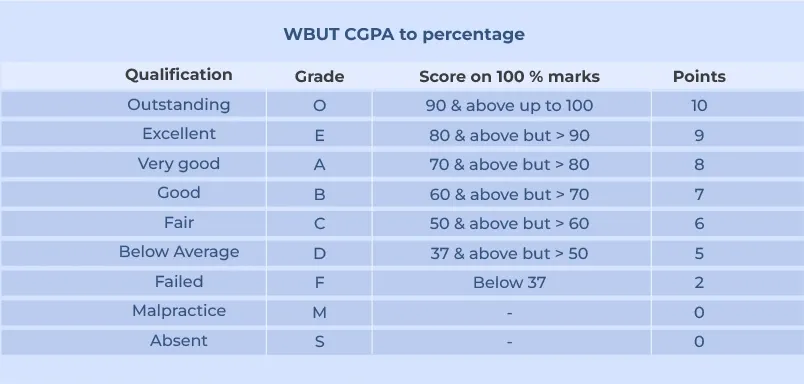WBUT doesn't have any specific formula for calculating the CGPA to percentage calculation. The assessment system followed here is a 10-point grade system. Thus the formula used will be as follows.

 WBUT CGPA To percentage = (CGPA-0.75)*10

### CGPA to percentage MG University Calculation

MG university CGPA to percentage calculation follows the basic percentage conversion. Here the CGPA value will be multiplied by 9.5 to arrive at the percentage.

CGPA to percentage MG University conversion = CGPA*9.5

### SRM CGPA to percentage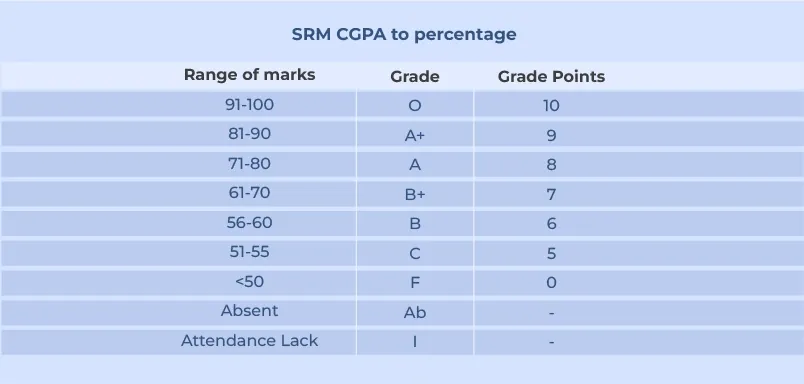The SRM students can use the following table to identify their grades obtained corresponding to their marks scored.

The overall CGPA will be calculated based on the individual semester Grade points obtained. Here the CGPA value differs between 0-10. Thus the percentage equivalence is calculated by multiplying the CGPA value by 10 approximately.

SRM CGPA to percentage= CGPA*10

### HNGU CGPA to percentage

In HNGU the CGPA to percentage calculation is done by simply multiplying the CGPA by 9.5.

Percentage = CGPA*9.5

### DU CGPA to percentage converter

Delhi University uses the Choice-Based Credit System (CBCS). Here the percentage from the CGPA is calculated using the usual formula of multiplying 9.5 by CGPA.

## How to calculate CGPA from percentage?

To calculate the CGPA from percentage, you have to just reverse the percentage calculation.

CGPA from percentage = Percentage / 9.5

For example,

If you have scored an 85%, then your CGPA will be,
CGPA= 85/9.5
= 8.95

If the percentage is 78, then the CGPA = 78/9.5 = 8.21

## FAQ on CGPA to percentage

### 1. When was the CGPA system introduced by the CBSC?

The CBSC has used the CGPA scoring method since the 2010 session. At first, the grading system was used only for 12th-grade students.

### 2. What is the percentage(%) of 7.6 CGPA?

To calculate the % of 7.6 CGPA, you need to multiply this value by 9.5. 7.6X9.5 = 72.2%

### 3. How to convert CGPA to percentage?

The basic formula for converting the CGPA into percentage is, by multiplying the CGPA by 9.5. However, each university may have its own calculation to arrive at the final percentage.

### 4. What is the CGPA to percentage calculator for Nagpur University?

Nagpur University uses the following formula to arrive at the percentage.

Percentage= (CGPA-0.75)*10

### 5. How to convert 8.4 CGPA to percentage?

Percentage= 8.4 * 9.5
= 79.8%

CGPA= 75/9.5
= 7.89

### 7. What is the maximum CGPA you can secure?

The CGPA ranges between 0.00 to 10.00 with 10 being the highest value. If you have received a 10 CGPA, then your percentage will be 95%.

#### Recent posts from NivethithaNivethitha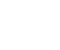Nivethitha

SGPA to PercentageNivethitha

CGPA to percentageNivethitha

Education loan in USA for Indian studentsNivethitha

Best place to study in CanadaNivethitha

IELTS Band ScoreA truly global higher education partner to learners and education institutions Support learners at every point in their global education journey with reliable services, products and solutions.

Kanan Int EdTech Inc

229, Yonge Street Suite 406 Toronto Ontario, Canada M5B 1N9

Kanan International Pvt. Ltd.+91 63570 12000admissions@kanan.co